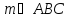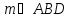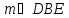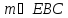# Unit 1 Lesson 4 Vertical Angles Exploration

 bet 1/2 Sana 16.12.2020 Hajmi 1.54 Mb.
1   2

## Unit 1 Lesson 4 Vertical Angles Exploration

This is an element of your Unit 1 Project and should be added to your Unit 1 Project portfolio after you answer the feedback on the assignment provided by your teacher.

## Essential Question:

• How can we use vertical angles to help build structures?

## Objectives:

• Write a theorem that can be used to solve problems involving vertical angles using the SPORT method.

• Solve problems involving vertical angles

## Part I: Specific Cases & Examples

Open the vertical angles GeoGebra applet on your laptop or iPad. Move the points D, E, and C around and notice how it changes the measures of all four angles. Record the measurements of the angles in 5 cases when it shows "Vertical Angles" on the screen and 5 cases where it does not show "Vertical Angles" on the screen. Include one screenshot of when you have vertical angles shown.
 Table 1: Showing "Vertical Angles" 5 Cases Case #1 2 3 4 5

 Table 2: NOT Showing "Vertical Angles" 5 Cases Case #1 2 3 4 5

 One Screenshot when Vertical Angles is shown:

1. In Table 1 above, name the two pairs of vertical angles:

1. In Table 1 above, name the angles that were adjacent, linear pairs: